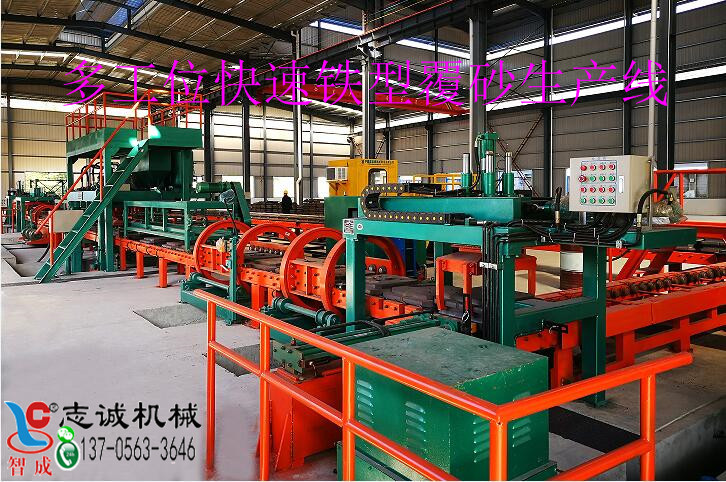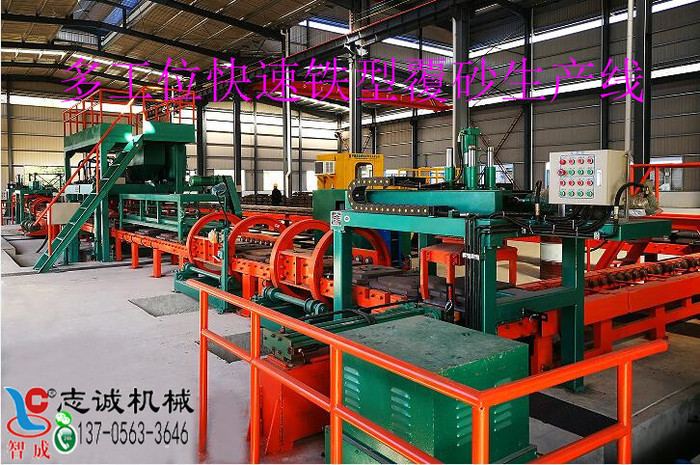# 選礦廠球磨機不同球徑裝球配比及實例(1)先取磨礦機全給礦樣(一般分別取新給礦和返砂樣，然后按比例配樣)逬行篩析，分成若干粒級，如分成18?12, 12?10, 10?8, 8?6等，稱重各粒級，算出各粒級質量百分比(產率)，如，，......等；

⑵用各級別粒度上限(如18、12、10、8......等)或上、下限

D10……

G重量=V有效×充填率×鋼球堆密度

①按公式計算出球的總質量G:

D-球磨機筒體內徑，m；

L-球磨機筒體有效長度，m;；

-介質充填率，%；

-球的堆密度，t/m3。

②依調整后的各級球比例計算出各級鋼球的質量分別為GD12=G.D12,GD10=G.D10,GD8=G.D8。

⑴礦磨機全給礦篩析結果見表1

 粒級（mm） 產率（%） 粒級（mm） 產率（%） 18 ?12 18-12=20 6 ?4 6-4=5 12 ?10 12-10=40 4 ?2 4-2=4 10 ?8 10-8=15 2 ?1 2-1=4 8?6 8-6=8 1 ?0 1-0=4

⑵按各級上限計算應配的球徑并取整，見表2

 粒級（mm） 球徑（mm） 粒級（mm） 球徑（mm） 18 ?12 D18=120 6 ?4 D6=70 12 ?10 D12=100 4 ?2 D4=60 10 ?8 D10=90 2 ?1 D2=50 8?6 D8=80 1 ?0 D1=40

⑶各種球的質量百分比見表3

 球徑（mm） 質量比列（%） 球徑（mm） 質量比列（%） 120 20 70 5 100 40 60 4 90 15 50 4 80 8 40 4

 球徑（mm） 質量比列（%） 球徑（mm） 質量比列（%） 120 30 80 13 100 45 60 12

⑷計算加入球的總質量G:

⑸計算各種球分別應加入質量

G120=G.120=11.928×0.3=3.85（t）

G100=G.100=11.928×0.45=5.37（t）

G80=G.80=11.928×0.13=1.55(t）

G60=G.60=11.928×0.12=1.43(t）